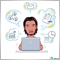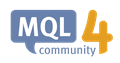# Getting data for different time periods12

Hello,

I want to get the chart data for, for example H1, M15 and M1 so that I can display something about them regardless of what time period is currently being displayed.

(I am very new to MT4, but not programming) *blushes*

I have seen an example where within OnCalculate, the flowing code is used to plot the open price, or other available data

```int uncalculatedBar = rates_total - prev_calculated;  // Get number of graph points to draw

int mVal = 0;

for (int i=0; i<uncalculatedBar; i++) {
Label1Buffer[i] = open[i];      //loop back through data plotting open price
}```

However, if I change the time period displayed, the loop here uses only that displayed data.

How would I get say the H1 data? I presume I can somehow set a period to get data from, but I am unsure where to start.226

RichMoo:

Hello,

I want to get the chart data for, for example H1, M15 and M1 so that I can display something about them regardless of what time period is currently being displayed.

(I am very new to MT4, but not programming) *blushes*

I have seen an example where within OnCalculate, the flowing code is used to plot the open price, or other available data

However, if I change the time period displayed, the loop here uses only that displayed data.

How would I get say the H1 data? I presume I can somehow set a period to get data from, but I am unsure where to start.